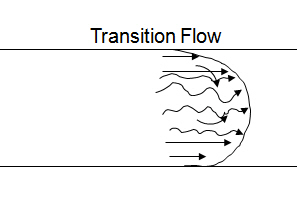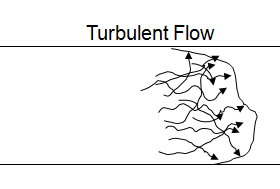## Pressure Drop through Surface Equipment

Pressure drop through surface equipment is one of the components in drilling hydraulics that must be considered. When we talk about surface equipment, we usually refer to those following equipment as a stand pipe, surface hoses, a swivel, a gooseneck and a Kelly.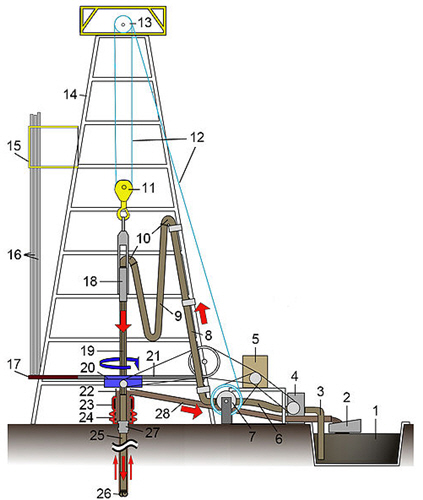Surface equipment on the rig – more detail here http://en.wikipedia.org/wiki/List_of_components_of_oil_drilling_rigs Continue reading

## Understand Pressure Loss (Frictional Pressure) in Drilling System

Frictional pressure is pressure loss acting in the opposite direction of fluid flow and today we will look into each component in pressure therefore you will get clearer picture about the frictional pressure in drilling system.

Let take a look at the simple diagram below.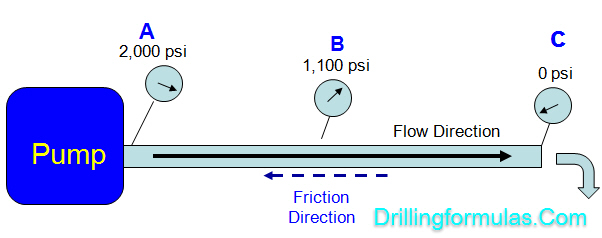A mud pump creates power to move drilling fluid from point A to C and the frictional pressure or pressure loss is the amount of pressure required to transfer fluid.

Pump pressure is 2,000 psi at the starting point (“A”) and at the end point (“C”), pressure is 0 psi. This tells you that you need 2,000 psi to overcome the frictional pressure in order to move the fluid from point “A” to point “C”.

“Differential pressure between two points in the system is pressure loss while fluid is moved from one to another point.”

## Pressure Drop Across a BitPressure across a bit occurs when the drilling mud passing through the jet nozzles. This pressure drop is important for drilling hydraulic optimization (maximum hydraulic horse power or impact force) and hole cleaning. The pressure drop at the nozzle area is a function of total flow area of the bit, flow rate and mud density.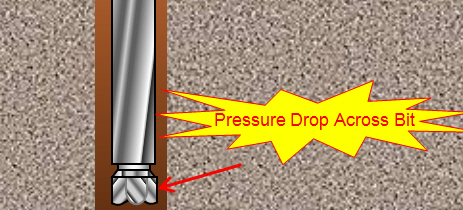Pressure drop across the bit can be determined by the following equation:

## Oilfield Unit

Pb = (Q²×W) ÷ (12031 × A²)

Where:

Pb = pressure drop across a bit, psi

Q = flow rate, gpm

W = mud weight, ppg

A = total flow area, square inch

Please use the following information to determine pressure drop across a bit

Flow rate = 800 gpm

Mud weight = 9.0 ppg

Total Flow Area = 0.3728 square inch

Pb = (800²×9.0) ÷ (12031 × 0.3728²)

Pb = 3,446 psi

## Metric Unit

Pb = (Q²×W) ÷ (7.191 × A²)

Where:

Pb = pressure drop across a bit, KPa

Q = flow rate, lpm

W = mud weight, kg/m³

A = total flow area, mm²

Please use the following information to determine pressure drop across a bit

Flow rate = 3,000 l/m

Mud weight = 1,100 kg/m³

Total Flow Area = 240 mm²

Pb = (3,000²×1,100) ÷ (7.191 × 240²)

Pb = 23,901  KPaRef books:

Lapeyrouse, N.J., 2002. Formulas and calculations for drilling, production and workover, Boston: Gulf Professional publishing.

Bourgoyne, A.J.T., Chenevert , M.E. & Millheim, K.K., 1986. SPE Textbook Series, Volume 2: Applied Drilling Engineering, Society of Petroleum Engineers.

Mitchell, R.F., Miska, S. & Aadny, B.S., 2011. Fundamentals of drilling engineering, Richardson, TX: Society of Petroleum Engineers.

## Type of Flow in Drilling Hydraulics

Flow of drilling fluids can be classified into one of three phases which are laminar, transition and turbulent flow.

Laminar Flow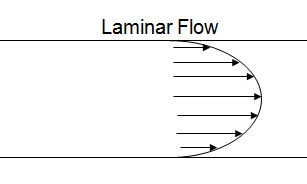Laminar flow, sometimes known as streamline flow, occurs when a fluid flows in parallel layers, with no disruption between the layers. At low velocities the fluid tends to flow without lateral mixing, and adjacent layers slide past one another like playing cards. There are no cross currents perpendicular to the direction of flow, nor eddies or swirls of fluids. In laminar flow the motion of the particles of fluid is very orderly with all particles moving in straight lines parallel to the pipe walls.

Transition FlowTransitional flow exhibits characteristics of both laminar and turbulent flow. The edges of the fluid flow in a laminar state, while the center of the flow remains turbulent. Like turbulent flows, transitional flows are difficult, if not impossible, to accurately measure.